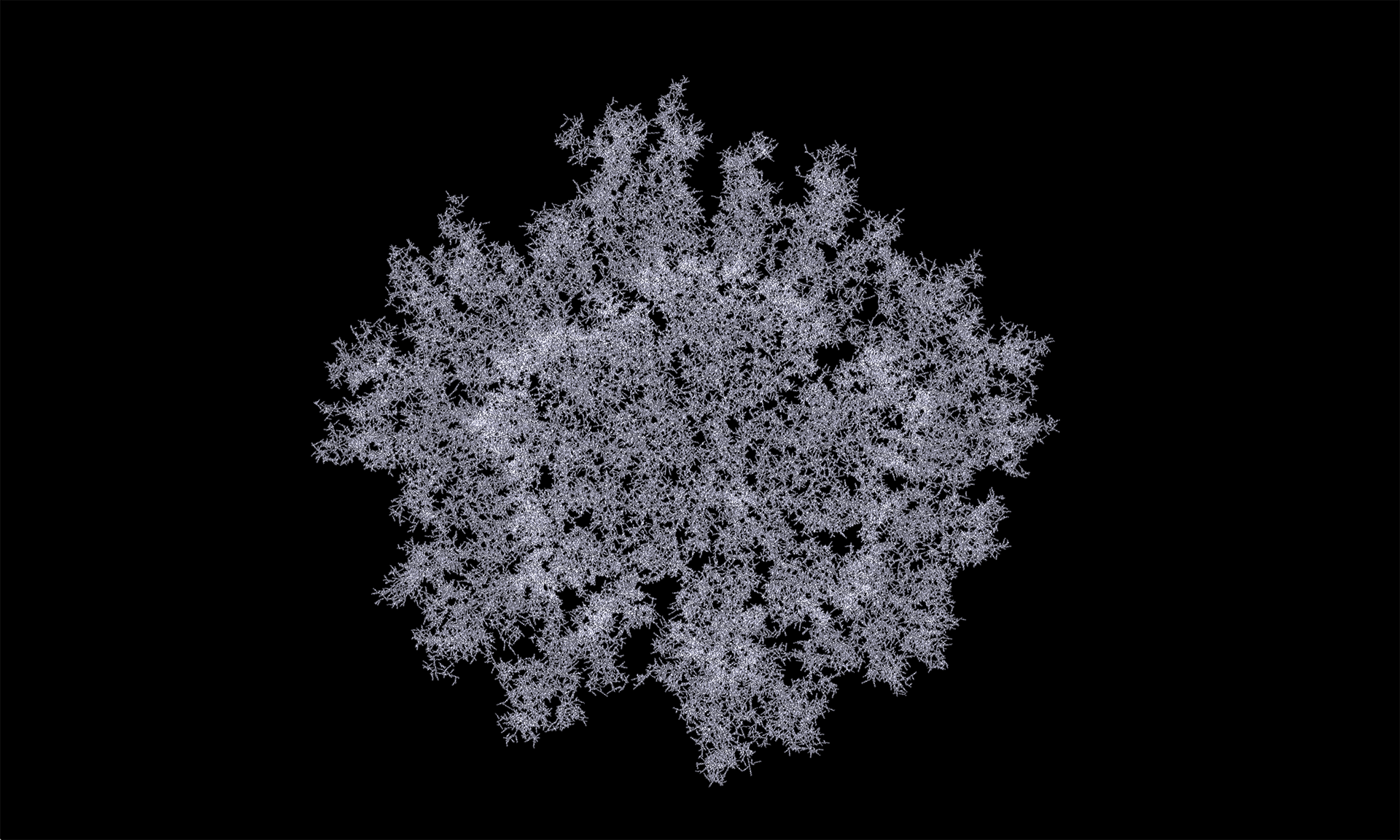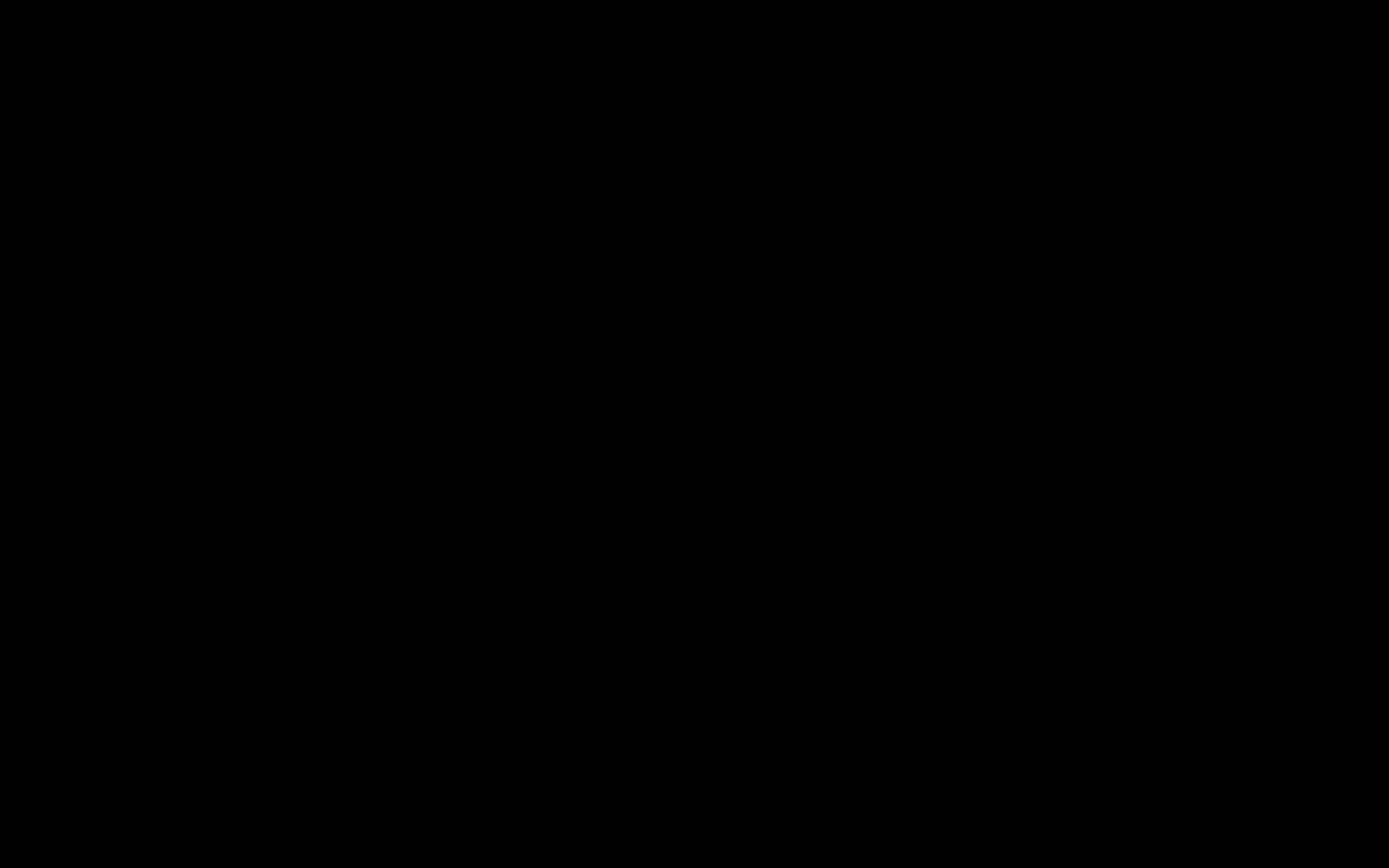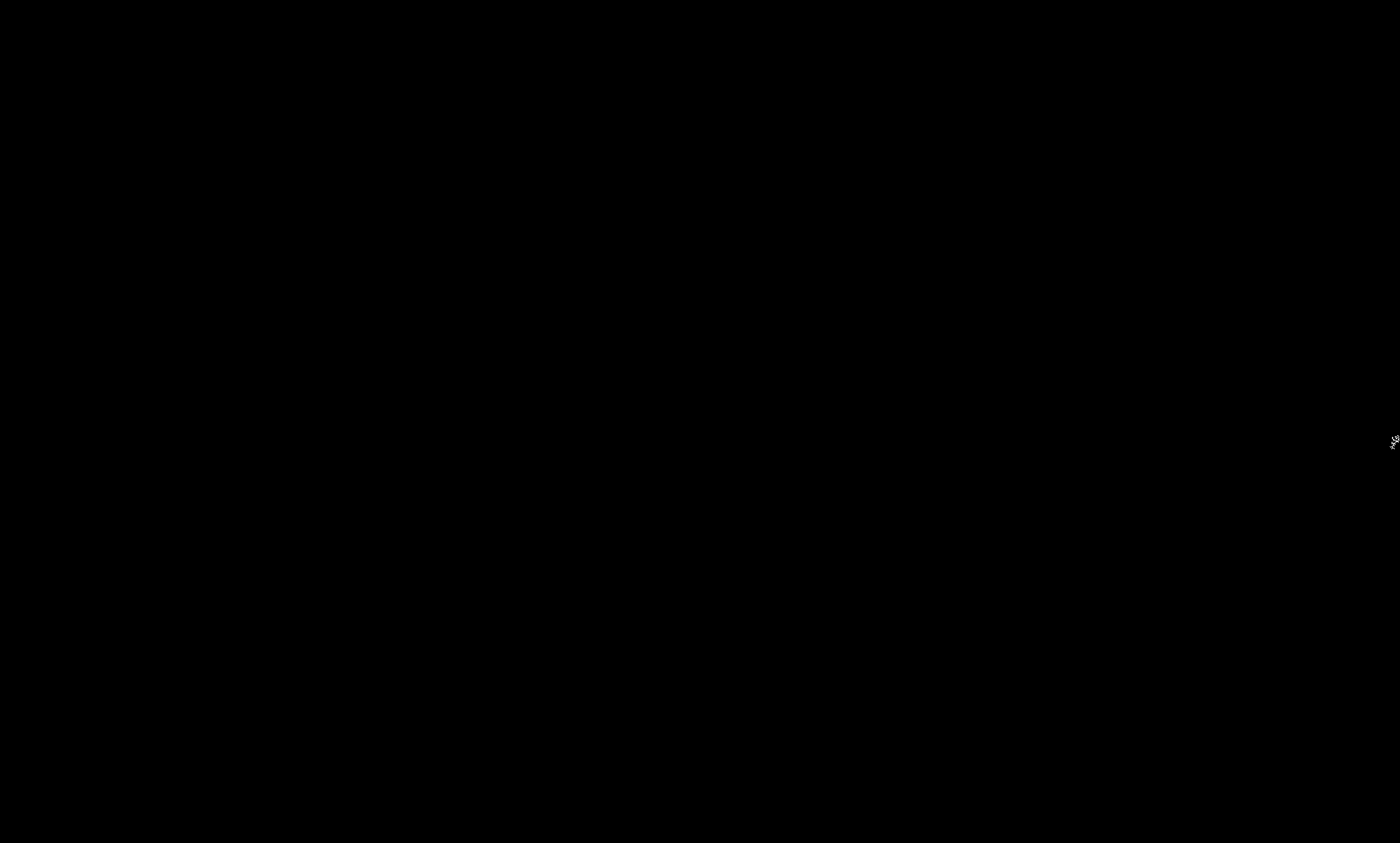# Diffusion Limited Aggregation

AUTHOR: Samuel Pietri
UPDATED:Diffusion limited aggregation is a technique that attempts to simulate certain forms of growth. It is interesting in that it is both very simple and has broad applications to many diverse phenomena. It has been used to model coral, dendritic crystals, lightning, and snowflakes.

This algorithm has been widely implemented in the digital/computational community, a few of these application are definitely worth watching such as the incredible simulations of Andy Lomas and Karsten Schmidt.

The premise is that you have particles moving randomly. This is called Brownian motion, which has an interesting history in its own right. When a particle hits a static structure, it sticks to it. In the most basic implementation, you start with a single fixed particle and add new particles in the manner described one at a time. The process naturally forms branches structures as the extremities of the growth act to block particles from hitting the interior parts of the static structure.

Pseudo Code:

``````Create a blank image
Plot a dot in the center
Repeat for a given number of particles:
Place particle randomly in the simulation
Repeat forever:
If particle is inside boundaries:
If any pixel around particle isn't blank:
Plot particle
Break forever loop
End If
Else
Limit particle's coordinates so it's inside the boundary
Plot particle
Break forever loop
End If
Move particle randomly
End Loop
End Loop

``````

Basic Implementation

There are two approaches to a basic DLA simulation. One works on a fixed grid and the other is grid-free and uses particles. This difference is reflected in many simulation techniques. Grids provide a rigid structure that simplifies the model. In this case a particle can only move along the grid to one of its four neighbours. Working without a grid provides more freedom, but generally creates additional complexity, which can mean it is harder to program or requires more computation.

In its simplest implementation the core function of the system is the one responsible for the detection of other neighbours in the surrounding area of a fixed particle. The first implementation involved a field array of pixels which can be either white (stuck) or black (alone) and for each pixel still active in the simulation I check its state.

``````bool aggParticle::alone(){
int cx = x;
int cy = y;

int lx = cx - 1;
int rx = cx + 1;
int ty = cy - 1;
int by = cy + 1;

if(cx <= 0 || cx >= w || lx <= 0 || lx >= w || rx <= 0 || rx >= w ||
cy <= 0 || cy >= h || ty <= 0 || ty >= h || by <= 0 || by >= h){
return true;
}

cy *= w;
by *= w;
ty *= w;

if(field[cx + ty] || field[lx + cy] || field[rx + cy] || field[cx + by]){
return false;
}

if(field[lx + ty] || field[lx + by] || field[rx + ty] || field[rx + by]){
return false;
}

return true;
}

``````

Note that you could run the simulation on any grid, though it would most likely require a slightly more complex data structure. You could even run it on arbitrary, irregular networks such as one formed from a voronoi diagram.

DLA simulation from a central source.In this case variability comes with the different position of the initial sources but on a general basis the particle’s motion logic is the main creative parameter to play with.

GLSL Implementation

The general algorithm becomes really slow when you scale things up. This is due the fact that neighbouring state search is done for each particle active in the simulation and involves distance calculation which are computationally expensive. To somehow avoid this issue I shifted my attention on a GLSL implementation, taking advantage of the GPU computation power when it comes to these kind of operations.

The initial set of particles still moves randomly in the screen and the stuck procudeure is written as follow:

``````float checkStuck(vec4 p, out vec4 dd, inout vec4 v, int idCount){

ivec2 texSize = textureSize(particles0);
float stuck;

for (int x = 0; x < texSize.x; x ++){
for (int y = 0; y < texSize.y; y++) {

vec3 pos = texture(particles0, vec2(x, y)).xyz;
float check = texture(particles0, vec2(x, y)).w;

if(check !=0){

float d = distance(p.xyz, pos);

//if the distance is > 0 and < dla radius
if (d > 0 && d < raddd ){

dd = vec4(p.xyz - pos, 1.0);
v.w = 0.0;
return check;

}
}

}

}

dd = vec4(0.0, 0.0, 0.0, 0.0);
return 0.0;
}

``````

Optimization

Generally, complex simulations will take a lot of computation, and depending on what you want to do with them speed can be an important factor. Therefore, optimising your algorithm is often a necessary step. It is not usually necessary to talk about low level optimization, such as reducing function calls or using fast bit level operations, but higher level algorithmic optimisation are important to understand.

….

Tags:

Categories: